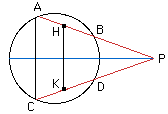Date: Tue, 18 Nov 1997 01:54:23 -0600 (CST)

Name: Herman

Who is asking: Student
Level: All

Question:
When produced, two equal chords AB and CD of a circle meet at P in an angle of 24 degrees. If H is the mid-point of AB and K is the mid-point of CD, calculate the size of angle HKD.

Hi HermanIf you draw the line from the midpoint of the segment AC to the mid point of the segment BD and extend it to meet AB extended, then the symmetry of the diagram tell you that these lines meet at P. The symmetry also implies that triangle APB is isosceles, and thus triangle HPK is isosceles and angle PHK = angle HKP= (180-24)/2 = 78 degrees.
Cheers,
Penny

Go to Math Central

To return to the previous page use your browser's back button.